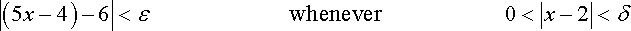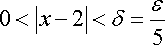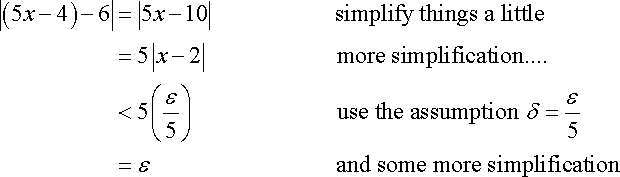# What is being Done in This proof of Limits?

I have been trying to learn calculus by my own, but when it comes to proving limits I get very confuse.

Could somebody explain me what is being done here?If you know any resources that could help me with this task let me know.

here is the source:
http://tutorial.math.lamar.edu/Classes/CalcI/DefnOfLimit.aspx

Last edited:

mfb
Mentor
The first line is the statement you want to show.
The second line is a clever guess for delta (as function of epsilon), and the remaining steps are just simplifications, showing that |(5x-4)-6| is indeed < epsilon if |x-2|<delta.

Mark44
Mentor
I have been trying to learn calculus by my own, but when it comes to proving limits I get very confuse.

Could somebody explain me what is being done here?If you know any resources that could help me with this task let me know.

here is the source:
http://tutorial.math.lamar.edu/Classes/CalcI/DefnOfLimit.aspx

They're starting with this inequality:
##|(5x - 4) - 6| < \epsilon##
In a few algebra operations, they arrive at this:
##5|x - 2| < \epsilon ##
or
##|x - 2| < \epsilon/5 ##
If you let ##\delta = \epsilon/5##, then by reversing the steps above, you'll get back to the first inequality.

The whole idea is sort of a challenge-response. If you're trying to convince someone that ##\lim_{x \to 2}5x -4 = 6##, they might ask you get a function value within 0.1 (that's the ##\epsilon##). You say, take any x within 0.1/5 = 0.02 of 2.

If the challenger isn't satisfied, he might ask if you can get the function value within 0.001. You tell him to take any x within 0.0002 of 2 (i.e., between 1.9998 and 2.0002).

And so on. Eventually, he'll give up and accept that the limit is indeed 2.

Last edited:
They're starting with this inequality:
##|(5x - 4) - 6| < \epsilon##
In a few algebra operations, they arrive at this:
##5|x - 2| < \epsilon ##
or
##|x - 2| < \epsilon/5 ##
If you let ##\delta = \epsilon/5##, then by reversing the steps above, you'll get back to the first inequality.

The whole idea is sort of a challenge-response. If you're trying to convince someone that ##\lim_{x \to 2}5x -4 = 6##, they might ask you get a function value within 0.1 (that's the ##\epsilon##). You say, take any x within 0.1/5 = 0.02 of 2.

If the challenger isn't satisfied, he might ask if you can get the function value within 0.001. You tell him to take any x within 0.0002 of 2 (i.e., between 1.9998 and 2.0002).

And so on. Eventually, he'll give up and accept that the limit is indeed 2.
thanks

Last edited by a moderator: